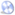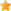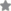### Finite Element Method with applications in EngineeringAuthor(s):
 Y. M. Desai T. I. Eldho A. H. Shah
Author: Y. M. Desai
• ISBN:9788131724644
• 10 Digit ISBN:8131724646
• Price:Rs. 650.00
• Pages:492
• Imprint:Pearson Education
• Binding:Paperback
• Status:Available
• With   CDRatings:This book presents a practical understanding of the finite element method with a variety of engineering applications that will aid students, teachers, practicing engineers and researchers. It begins with an introduction to the mathematical modeling of engineering problems and approximate methods of analysis. It then introduces the different approaches in FEM such as direct approach, principle of virtual work, variational principle and method of weighted residual. Finally, the applications of FEM to real-world problems are presented in 1D, 2D and 3D for structural analysis, heat and mass transfer, geo-mechanical, fluid flow and other problems.

### Table of Content

1. Introduction
2. Approximate Methods of Analysis
3. Finite Element Method—An Introduction
4. Different Approaches in FEM
5. Finite Elements and Interpolation Functions
6. One-Dimensional Finite Element Analysis
7. Two-Dimensional Finite Element Analysis
8. Three-Dimensional Finite Element Analysis
9. Computer Implementation of FEM
10. Further Applications of Finite Element Method

11. Appendix A Review of Matrix Algebra and Matrix Calculus
Appendix B Elements of Calculus of Variations
Appendix C Example Illustrating Use of Galerkin’s Method
Appendix D Review of Gauss Quadrature Procedure for Numerical Integration
Appendix E User’s Manual for the Simplified Finite Element Analysis Program (sfeap)
Appendix F Graphical Interface for the Simplified Finite Element Analysis Program (sfeap)
Appendix G Computer Programs for One-Dimensional and Two-Dimensional Problems
Index

### Salient Features

• Separate chapters are devoted to basic mathematical modeling, approximate method of analysis, introduction and different approaches to FEM
• Comprehensive coverage of FEM interpolation functions
• Finite element analysis for various problems in 1D, 2D and 3D
• Comprehensive coverage of computer implementation of FEM and FEM software and web resources
• Large number of solved problems and exercise questions
• More than 200 figures for better understanding of the concepts